# A thin steel wire of uniform cross section is bent into the shape shown. Locate its center of gravity.

Question-AnswerCategory: Engineering MechanicsA thin steel wire of uniform cross section is bent into the shape shown. Locate its center of gravity.

A thin steel wire of uniform cross section is bent into the shape shown. Locate its center of gravity.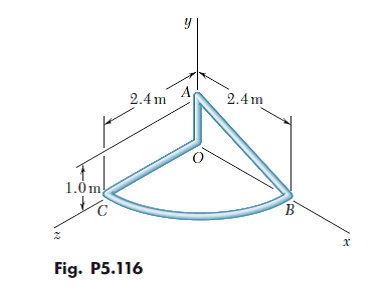Step: 1

Draw the free body diagram of line segments.Step: 2

The lengths and coordinate of the centroid of each of these components are entered in table below.

Line segment

Length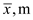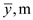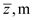OA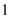0

0.5

0

AB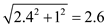1.2

0.5

0

BC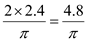0CO

2.4

0

0

1.2Step: 3

Calculate the first moment of its length with respect to the yz plane: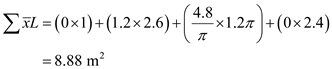Calculate the first moment of its length with respect to the xz plane: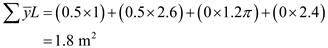Calculate the first moment of its length with respect to the xy plane: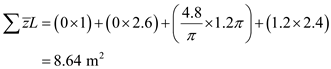Step: 4

Calculate the x – coordinate of the body:Substitute,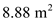for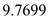forTherefore, the x – coordinate of the centre of gravity is.

Step: 5

Calculate the y – coordinate of the body: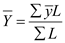Substitute,for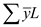for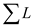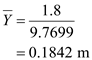Therefore, the y – coordinate of the centre of gravity is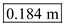.

Step: 6

Calculate the z – coordinate of the body: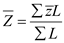Substitute,forforTherefore, the z – coordinate of the centre of gravity is.